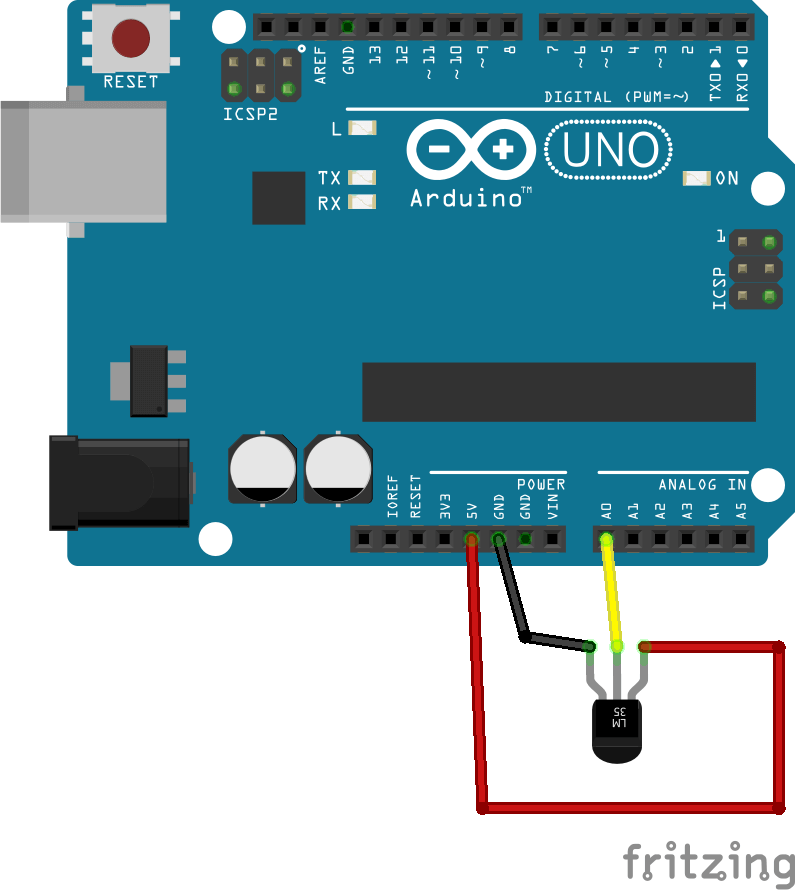Select Page
Tags: , , ,
0
(0)

The temperature sensor LM35 is a small easy to use, easy to find device. Its output voltage is directly proportional to the temperature and gives a precise measurement.

## Material

• Computer
• Arduino UNO
• USB cable to connect Arduino to the computer
• Temperature sensor LM35

## Wiring## Code

The datasheet of the sensor LM35 gives the temperature range measurement, -55 to 150°C , and the conversion rule between voltage and temperature value, 10 mV/°C. The value is read with an analog port of the Arduino, so the output voltage (0 to 5V) is converted on a digital value (0 to 1023).

```// Constants
#define DELAY 1000 // Delay between two measurements in ms

// Parameters
const int sensorPin = A0; // Pin connected to sensor

// Variables
float voltage, temperature;

void setup(void) {
Serial.begin(9600);
}

void loop(void) {
voltage= analogRead(sensorPin) * (5.0 / 1023.0); // Convert digital value to voltage
Serial.print("Sensor voltage = ");
temperature=100*voltage; // conversion from V to °C

delay(DELAY);
}

```

## Source

Find other examples and tutorials in our Automatic code generator

Click on a star to rate it!

Average rating 0 / 5. Vote count: 0

No votes so far! Be the first to rate this post.

As you found this post useful...